## jueves, 7 de marzo de 2013

### A* Seacrh algorithm

A* uses a best-first search and finds a least-cost path from a given initial node to one goal node (out of one or more possible goals). As A* traverses the graph, it follows a path of the lowest expected total cost or distance, keeping a sorted priority queue of alternate path segments along the way.

It uses a knowledge-plus-heuristic cost function of node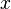(usually denoted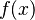) to determine the order in which the search visits nodes in the tree. The cost function is a sum of two functions:
• the past path-cost function, which is the known distance from the starting node to the current node$x$ (usually denoted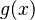$g(x)$)
• a future path-cost function, which is an admissible "heuristic estimate" of the distance from$x$ to the goal (usually denoted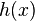$h(x)$).
The$h(x)$ part of the$f(x)$ function must be an admissible heuristic; that is, it must not overestimate the distance to the goal. Thus, for an application like routing,$h(x)$ might represent the straight-line distance to the goal, since that is physically the smallest possible distance between any two points or nodes.

## Pseudocode

```function A*(start,goal)
closedset := the empty set    // The set of nodes already evaluated.
openset := {start}    // The set of tentative nodes to be evaluated, initially containing the start node
came_from := the empty map    // The map of navigated nodes.

g_score[start] := 0    // Cost from start along best known path.
// Estimated total cost from start to goal through y.
f_score[start] := g_score[start] + heuristic_cost_estimate(start, goal)

while openset is not empty
current := the node in openset having the lowest f_score[] value
if current = goal
return reconstruct_path(came_from, goal)

remove current from openset
for each neighbor in neighbor_nodes(current)
tentative_g_score := g_score[current] + dist_between(current,neighbor)
if neighbor in closedset
if tentative_g_score >= g_score[neighbor]
continue

if neighbor not in openset or tentative_g_score < g_score[neighbor]
came_from[neighbor] := current
g_score[neighbor] := tentative_g_score
f_score[neighbor] := g_score[neighbor] + heuristic_cost_estimate(neighbor, goal)
if neighbor not in openset

return failure

function reconstruct_path(came_from, current_node)
if came_from[current_node] in set
p := reconstruct_path(came_from, came_from[current_node])
return (p + current_node)
else
return current_node```
` `

## Complexity

The time complexity of A* depends on the heuristic. In the worst case, the number of nodes expanded is exponential in the length of the solution (the shortest path), but it is polynomial when the search space is a tree, there is a single goal state, and the heuristic function h meets the following condition: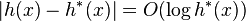$|h(x) - h^*(x)| = O(\log h^*(x))$
where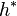$h^*$ is the optimal heuristic, the exact cost to get from$x$ to the goal.

from wikipedia
` `

## martes, 31 de enero de 2012

Begins at the root node and explores all the neighboring nodes. Then for each of those nearest nodes, it explores their unexplored neighbor nodes, and so on, until it finds the goal.
Order in which the nodes are expanded

BFS is an uninformed search method that aims to expand and examine all nodes of a graph or combination of sequences by systematically searching through every solution. In other words, it exhaustively searches the entire graph or sequence without considering the goal until it finds it. It does not use a heuristic algorithm.

From the standpoint of the algorithm, all child nodes obtained by expanding a node are added to a FIFO (i.e., First In, First Out) queue. In typical implementations, nodes that have not yet been examined for their neighbors are placed in some container (such as a queue or linked list) called "open" and then once examined are placed in the container "closed".

## Algorithm

1. Enqueue the root node
2. Dequeue a node and examine it
• If the element sought is found in this node, quit the search and return a result.
• Otherwise enqueue any successors (the direct child nodes) that have not yet been discovered.
3. If the queue is empty, every node on the graph has been examined – quit the search and return "not found".
4. If the queue is not empty, repeat from Step 2.

Note: Using a stack instead of a queue would turn this algorithm into a depth-first search.

## Pseudocode

Input: A graph G and a root v of G

`1  procedure BFS(G,v):2      create a queue Q3      enqueue v onto Q4      mark v5      while Q is not empty:6          t ← Q.dequeue()7          if t is what we are looking for:8              return t9          for all edges e in G.incidentEdges(t) do10             o ← G.opposite(t,e)11             if o is not marked:12                  mark o13                  enqueue o onto Q`

## lunes, 30 de enero de 2012

### Depth- first search (DFS)

Starts at the root (selecting some node as the root in the graph case) and explores as far as possible along each branch before backtracking.

Order in which the nodes are visited

DFS is an uninformed search that progresses by expanding the first child node of the search tree that appears and thus going deeper and deeper until a goal node is found, or until it hits a node that has no children. Then the search backtracks, returning to the most recent node it hasn't finished exploring. In a non-recursive implementation, all freshly expanded nodes are added to a stack for exploration.

Worst case performance
: O( | V | + | E | ) for explicit graphs traversed without repetition, O(bd) for implicit graphs with branching factor b searched to depth d.

Worst case space complexity: O( | V | ) if entire graph is traversed without repetition, O(longest path length searched) for implicit graphs without elimination of duplicate nodes.

## Pseudocode

Input: A graph G and a vertex v of G

Output: A labeling of the edges in the connected component of v as discovery edges and back edges

`1  procedure DFS(G,v):2      label v as explored3      for all edges e in G.incidentEdges(v) do4          if edge e is unexplored then5              w ← G.opposite(v,e)6              if vertex w is unexplored then7                  label e as a discovery edge8                  recursively call DFS(G,w)9          else10             label e as a back edge`

## domingo, 29 de enero de 2012

### partial plan space search

Partial plan space search uses a Backward inference search from the goal.

Each node of the search space is a partial plan

It establishes diferent types of constraints, for example, precedence constraint (a must precede b), binding constraints (inequality constraints, e.g., v1 ≠ v2 or v ≠ c or equality constraints (e.g., v1 = v2 or v = c) and causal link (use action a to establish the precondition p needed by action b).

It makes more and more refinements, until it has a solution.

POPLAN, TOPLAN and SATPlan.

### non-STRIPS style planning

• based on Planning Graph structure.
• based on propositional logic : Pushing the Envelope: Planning, Propositionl Logic, and Stochastic Search

based on Planning Graph structure:

• Graphplan (Fast Planning Through Planning Graph Analysis): A Planning Graph encodes the planning problem in such a way that many useful constraints inherent in the problem become explicitly available to reduce the amount of search needed. Furthermore, Planning Graphs can be constructed quickly: they have polynomial size and can be built in polynomial time.

A Planning Graph is not the state-space graph. In fact, unlike the state-space graph in which a plan is a path through the graph, in a Planning Graph a plan is essentially a flow in the network flow sense.

Searching for a plan: Given a Planning Graph, Graphplan searches for a valid plan using a backward-chaining strategy. It uses a level-by-level approach, in order to best make use of the mutual exclusion constraints.

In particular, given a set of goals at time t, it attempts to find a set of actions (no-ops included) at time t − 1 having these goals as add effects. The preconditions to these actions form a set of subgoals at time t − 1 having the property that if these goals can be achieved in t − 1 steps, then the original goals can be achieved in t steps. If the goal set at time t − 1 turns out not to be solvable, it tries to find a different set of actions, continuing until it either succeeds or has proven that the original set of goals is not solvable at time t.

To implement this strategy, it uses the following recursive search method:

For each goal at time t in some arbitrary order, select some action at time t − 1 achieving that goal that is not exclusive of any actions that have already been selected. Continue recursively with the next goal at time t. (Of course, if by good fortune a goal has already been achieved by some previously-selected action, we do not need to select a new action for it.) If our recursive call returns failure, then try a different action achieving our current goal, and so forth, returning failure once all such actions have been tried. Once finished with all the goals at time t, the preconditions to the selected actions make up the new goal.

## viernes, 29 de julio de 2011

### Coordination in multi-agent systems

To make coordination in multi-agent systems, we have to identify several characteristics. First, we have to identify the common problems that underlie any coordination situation. then we have to choose the mechanism to solve the coordination problem, a general coodination mechanisms that can be applied to a variety of situations. Finally, we have to focus in the applicability and usability of a given coordination mechanism. Identify the factors determining the applicability of the coordination mechanism. For instance, the numbe of agents in the system environment or the rate of change in the environment.

Identifing the common problems or reasons to any coordination situation:

[1 Jennings, 1996] and [2 Nwana, 1996] give many reasons that might necessitate coordination in multi-agent systems. The more important are:
• Meeting global constraints: If there are global budget limits, then agents must make agreements.
• Distributed information, expertise or resources: Often, a task cannot be performed by a single agent alone. If the required capabilities are distributed among the agents, coordination is necessary.
• Dependencies between the agents' actions: For instance, different tasks may need the same resources or there may exist a precedence relation between tasks.
The coordination can be seen of the point of view of an individual agent [3 Decker and Lesser, 1994]. Based on the definition of coordination given by [1 y 2], that is, that coordination is the act of managing interdependencies between activities.  say that there is a coordination problem if one of the following conditions is met:
• The actions agents affects the performance.
• The order of the actions affects performance.
• The execution time affects the performance.
We can also find general coordination processes that occur in many domains:
• Coordinated goal selection.
• Coordinated resource allocation.
• Coordinated sequencing (one activity after the other) and synchronizing (activities at the same time).
[5 Durfe, 2001] concludes that the applicability of every coordination mechanism has its limits:

It does not possible, however to devise a coordination strategy that works well under all circumstances; whatever strategy we adopt, certain situations can stress it to the breaking point.

 Identifies three varying properties (dimensions) of an interaction situation: The agent population, the task environment and the solution propierties.

The most obvios properties that impact the usability of a coordination strategy are:

Agent population: Quantity, the number of agents. Heterogeneity, agents can have different capabilities, internal architectures and communication languages. Complexity, how predictable the agents are.

Task environment: Degree of interaction, large and small groups of agents. Dynamics, The rate at which the environment changes. Distributivity, tasks can originate centrally or distributively.

Solution properties: Quality, the quality of a solution by judging how well it coordinates agent interactions (how efficient it is utilizing agent resources). Robustness, changes in the environment invalidate the plans or goals of agents. Overhead limitations, communication bandwidth may be limited.